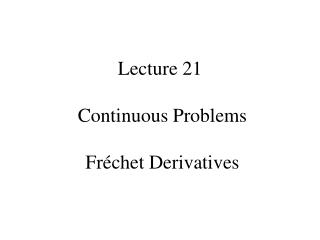DownloadDownload PresentationLecture 21 Continuous Problems Fr é chet Derivatives

Lecture 21 Continuous Problems Fr é chet Derivatives

Télécharger la présentationLecture 21 Continuous Problems Fr é chet Derivatives

- - - - - - - - - - - - - - - - - - - - - - - - - - - E N D - - - - - - - - - - - - - - - - - - - - - - - - - - -
Presentation Transcript

1. Lecture 21 Continuous ProblemsFréchet Derivatives

2. Syllabus Lecture 01 Describing Inverse ProblemsLecture 02 Probability and Measurement Error, Part 1Lecture 03 Probability and Measurement Error, Part 2 Lecture 04 The L2 Norm and Simple Least SquaresLecture 05 A Priori Information and Weighted Least SquaredLecture 06 Resolution and Generalized Inverses Lecture 07 Backus-Gilbert Inverse and the Trade Off of Resolution and VarianceLecture 08 The Principle of Maximum LikelihoodLecture 09 Inexact TheoriesLecture 10 Nonuniqueness and Localized AveragesLecture 11 Vector Spaces and Singular Value Decomposition Lecture 12 Equality and Inequality ConstraintsLecture 13 L1 , L∞ Norm Problems and Linear ProgrammingLecture 14 Nonlinear Problems: Grid and Monte Carlo Searches Lecture 15 Nonlinear Problems: Newton’s Method Lecture 16 Nonlinear Problems: Simulated Annealing and Bootstrap Confidence Intervals Lecture 17 Factor AnalysisLecture 18 Varimax Factors, Empircal Orthogonal FunctionsLecture 19 Backus-Gilbert Theory for Continuous Problems; Radon’s ProblemLecture 20 Linear Operators and Their AdjointsLecture 21 Fréchet DerivativesLecture 22 Exemplary Inverse Problems, incl. Filter DesignLecture 23 Exemplary Inverse Problems, incl. Earthquake LocationLecture 24 Exemplary Inverse Problems, incl. Vibrational Problems

3. Purpose of the Lecture • use adjoint methods to compute • data kernels

4. Part 1 • Review of Last Lecture

5. a functionm(x) is the continuous analog of a vector m

6. a linear operatorℒis the continuous analog of a matrix L

7. a inverse of a linear operatorℒ-1is the continuous analog of the inverse of a matrix L-1

8. a inverse of a linear operatorcan be used to solvea differential equationif ℒm=f then m=ℒ-1fjust as the inverse of a matrixcan be used to solvea matrix equation if Lm=f then m=L-1f

9. the adjoint of a linear operatoris the continuous analog of the transpose of a matrix LT ℒ †

10. the adjoint can be used tomanipulate an inner productjust as the transpose can be used to manipulate the dot product(La) Tb=a T(LTb) (ℒa, b) =(a, ℒ†b)

11. table of adjoints c(x) • d/dx • d2/dx2 c(x) • -d/dx • d2/dx2

12. Part 2 • definition of the Fréchet derivatives

13. first rewrite the standard inverse theory equation in terms of perturbations a small change in the model causes a small change in the data

14. second compare with the standard formula for a derivative

15. third identify the data kernel asa kind of derivative thiskind of derivative is called a • Fréchet derivative

16. definition of a Fréchet derivative , this is mostlylingo though perhaps it adds a little insight about what the data kernel is

17. Part 2 • Fréchet derivative of Error

18. treat the data as a continuous function d(x) • then the standard L2 norm error is

19. = • let the data d(x) be related to the model m(x) • by • could be the data kernel integral • because integrals are linear operators

20. now do a little algebra to relate • to • a perturbation in the model • causes • a perturbation in the error

21. ifm(0)implies d(0)with error E(0)then ...

22. ifm(0)implies d(0)with error E(0)then ... all this is just algebra

23. ifm(0)implies d(0)with error E(0)then ... • use δd = ℒδm

24. ifm(0)implies d(0)with error E(0)then ... • use adjoint

25. ifm(0)implies d(0)with error E(0)then ... • Fréchet derivative of Error

26. you can use this derivative to solve and inverse problem using thegradient method

27. example

28. example d(x) = this is the relationship between model and data

30. example • Fréchet derivative of Error

31. (A) m(x) (B) x d(x) (C) x log10 E/E1 iteration

32. Part 3 • Backprojection

33. continuous analog of least squares

34. now define the identity operator ℐ m(x) = ℐ m(x)

35. view as a recursion

36. view as a recursion using the adjoint as if it were the inverse

37. example exact • m(x) = ℒ-1 dobs = ddobs / dx backprojection

38. example exact • m(x) = ℒ-1 dobs = ddobs / dx backprojection crazy!

39. (A) m(x)true x (B) mest(x) x (C) m(1)(x) x

40. interpretation as tomography m is slowness d is travel time of a ray from –∞ to x backprojection integrate (=add together) the travel times of all rays that pass through the point x

41. discrete analysisGm=d G= UΛVTG-g= VΛ-1UT GT=VΛUT ifΛ-1≈ ΛthenG-g≈GT backprojection works when the singular values are all roughly the same size

42. suggests scaling Gm=d → WGm=Wd where W is a diagonal matrix chosen to make the singular values more equal in overall size Traveltime tomography: Wii = (length of ith ray)-1 so [Wd]ihas interpretation of the average slowness along the ray i. Backprojection now adds together the average slowness of all rays that interact with the point x.

43. (A) (B) (C) x x x y y y

44. Part 4 • Fréchet Derivative • involving a differential equation

45. Part 4 • Fréchet Derivative • involving a differential equation seismic wave equation Navier-Stokes equation of fluid flow etc

46. data d is related to field u via an inner product field u is related to model parameters m via a differential equation

47. pertrubationδd is related to perturbation δu via an inner product write in terms of perturbations • perturbation δu is related to perturbation δm via a differential equation

48. what’s the data kernel ?

49. easy using adjoints data inner product with field